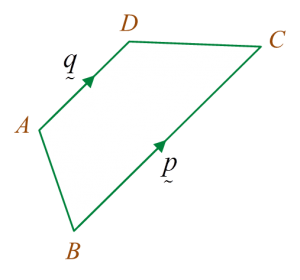\

# SPM Additional Mathematics 2017, Paper 1 (Question 4 – 7)

Question 4 (3 marks):
Diagram 4 shows a trapezium ABCD.Diagram 4

Solution:

Question 5 (3 marks):

Solution:
$\begin{array}{l}\frac{{25}^{h+3}}{{125}^{p-1}}=1\\ {25}^{h+3}={125}^{p-1}\\ {\left({5}^{2}\right)}^{h+3}={\left({5}^{3}\right)}^{p-1}\\ {5}^{2h+6}={5}^{3p-3}\\ 2h+6=3p-3\\ 3p=2h+9\\ p=\frac{2h+9}{3}\end{array}$

Question 6 (3 marks):

Solution:

Question 7 (2 marks):
It is given that the nth term of a geometric progression is
State
(a) the value of k,
(b) the first term of progression.

Solution:
(a)
k = 0, k = 1 or k = -1 (Any one of these answer).

(b)